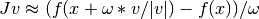# scipy.optimize.newton_krylov¶

scipy.optimize.newton_krylov(F, xin, iter=None, rdiff=None, method='lgmres', inner_maxiter=20, inner_M=None, outer_k=10, verbose=False, maxiter=None, f_tol=None, f_rtol=None, x_tol=None, x_rtol=None, tol_norm=None, line_search='armijo', callback=None, **kw)

Find a root of a function, using Krylov approximation for inverse Jacobian.

This method is suitable for solving large-scale problems.

Parameters : F : function(x) -> f Function whose root to find; should take and return an array-like object. x0 : array-like Initial guess for the solution rdiff : float, optional Relative step size to use in numerical differentiation. method : {‘lgmres’, ‘gmres’, ‘bicgstab’, ‘cgs’, ‘minres’} or function Krylov method to use to approximate the Jacobian. Can be a string, or a function implementing the same interface as the iterative solvers in scipy.sparse.linalg. The default is scipy.sparse.linalg.lgmres. inner_M : LinearOperator or InverseJacobian Preconditioner for the inner Krylov iteration. Note that you can use also inverse Jacobians as (adaptive) preconditioners. For example, >>> jac = BroydenFirst() >>> kjac = KrylovJacobian(inner_M=jac.inverse).  If the preconditioner has a method named ‘update’, it will be called as update(x, f) after each nonlinear step, with x giving the current point, and f the current function value. inner_tol, inner_maxiter, ... : Parameters to pass on to the “inner” Krylov solver. See scipy.sparse.linalg.gmres for details. outer_k : int, optional Size of the subspace kept across LGMRES nonlinear iterations. See scipy.sparse.linalg.lgmres for details. iter : int, optional Number of iterations to make. If omitted (default), make as many as required to meet tolerances. verbose : bool, optional Print status to stdout on every iteration. maxiter : int, optional Maximum number of iterations to make. If more are needed to meet convergence, NoConvergence is raised. f_tol : float, optional Absolute tolerance (in max-norm) for the residual. If omitted, default is 6e-6. f_rtol : float, optional Relative tolerance for the residual. If omitted, not used. x_tol : float, optional Absolute minimum step size, as determined from the Jacobian approximation. If the step size is smaller than this, optimization is terminated as successful. If omitted, not used. x_rtol : float, optional Relative minimum step size. If omitted, not used. tol_norm : function(vector) -> scalar, optional Norm to use in convergence check. Default is the maximum norm. line_search : {None, ‘armijo’ (default), ‘wolfe’}, optional Which type of a line search to use to determine the step size in the direction given by the Jacobian approximation. Defaults to ‘armijo’. callback : function, optional Optional callback function. It is called on every iteration as callback(x, f) where x is the current solution and f the corresponding residual. sol : array-like An array (of similar array type as x0) containing the final solution. NoConvergence : When a solution was not found.

Notes

This function implements a Newton-Krylov solver. The basic idea is to compute the inverse of the Jacobian with an iterative Krylov method. These methods require only evaluating the Jacobian-vector products, which are conveniently approximated by numerical differentiation:Due to the use of iterative matrix inverses, these methods can deal with large nonlinear problems.

Scipy’s scipy.sparse.linalg module offers a selection of Krylov solvers to choose from. The default here is lgmres, which is a variant of restarted GMRES iteration that reuses some of the information obtained in the previous Newton steps to invert Jacobians in subsequent steps.

For a review on Newton-Krylov methods, see for example [KK], and for the LGMRES sparse inverse method, see [BJM].

References

 [KK] (1, 2) D.A. Knoll and D.E. Keyes, J. Comp. Phys. 193, 357 (2003).
 [BJM] (1, 2) A.H. Baker and E.R. Jessup and T. Manteuffel, SIAM J. Matrix Anal. Appl. 26, 962 (2005).

#### Previous topic

scipy.optimize.broyden2

#### Next topic

scipy.optimize.anderson